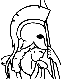#Stochastic Optimal Control: The Discrete-Time Case Preface:

This monograph is the outgrowth of research carried out at the University of Illinois over a three-year period beginning in the latter half of 1974. The objective of the monograph is to provide a unifying and mathematically rigorous theory for a broad class of dynamic programming and discrete-time stochastic optimal control problems. It is divided into two parts, which can be read independently.

Part I provides an analysis of dynamic programming models in a unified framework applicable to deterministic optimal control, stochastic optimal control, minimax control, sequential games, and other areas. It resolves the structural questions associated with such problems, i.e., it provides results that draw their validity exclusively from the sequential nature of the problem. Such results hold for models where measurability of various objects is of no essential concern, for example, in deterministic problems and stochastic problems defined over a countable probability space. The starting point for the analysis is the mapping defining the dynamic programming algorithm. A single abstract problem is formulated in terms of this mapping and counterparts of nearly all results known for deterministic optimal control problems are derived. A new stochastic optimal control model based on outer integration is also introduced in this part. It is a broadly applicable model and requires no topological assumptions. We show that all the results of Part I hold for this model.

Part II resolves the measurability questions associated with stochastic optimal control problems with perfect and imperfect state information. These questions have been studied over the past fifteen years by several researchers in statistics and control theory. As we explain in Chapter 1, the approaches that have been used are either limited by restrictive assumptions such as compactness and continuity or else they are not sufficiently powerful to yield results that are as strong as their structural counterparts. These deficiencies can be traced to the fact that the class of policies considered is not sufficiently rich to ensure the existence of everywhere optimal or epsilon-optimal policies except under restrictive assumptions. In our work we have appropriately enlarged the space of admissible policies to include universally measurable policies. This guarantees the existence of epsilon-optimal policies and allows, for the first time, the development of a general and comprehensive theory which is as powerful as its deterministic counterpart.

We mention, however, that the class of universally measurable policies is not the smallest class of policies for which these results are valid. The smallest such class is the class of limit measurable policies discussed in Section 11.1. The sigma-algebra of limit measurable sets (or C-sets) is defined in a constructive manner involving transfinite induction that, from a set of theoretic point of view, is more satisfying than the definition of the universal sigma-algebra. We believe, however, that the majority of readers will find the universal sigma-algebra and the methods of proof associated with it more understandable, and so we devote the main body of Part II to models with universally measurable policies.

Parts I and II are related and complement each other. Part II makes extensive use of the results of Part I. However, the special forms in which these results are needed are also available in other sources (e.g., the textbook by Bertsekas [B4]). Each time we make use of such a result, we refer to both Part I and the Bertsekas textbook, so that Part II can be read independently of Part I. The developments in Part II show also that stochastic optimal control problems with measurability restrictions on the admissible policies can be embedded within the framework of Part I, thus demonstrating the broad scope of the formulation given there.